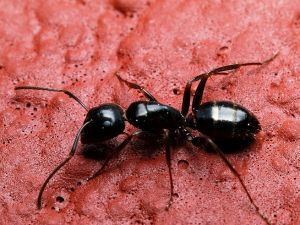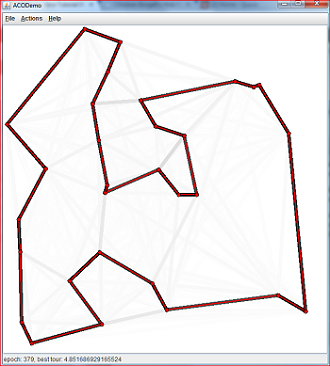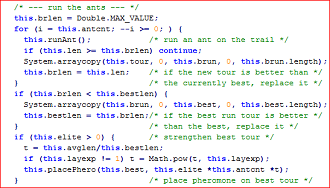# Ant Colony Optimization in Java

Long back I introduced Wordle word clouds as part of Java gallery and then completely forgot about the gallery category. Today I was reading about ant colony optimization and came across a nice implementation of it in Java. Thought of sharing this Java application to you as part of Java gallery.

Ant colony optimization is an awesome algorithm inspired by ant’s natural intelligence. Like cockroaches, ants are extremely successful insects surviving for millions of years. Ants live in colonies and they have hierarchies among them. Physical castes are, like worker ants have responsibilities divided based on their size.Ants communicate within themselves effectively. Their form of communication is efficient enough to help them survive for millions of years. Apart from sound, touch they use a secreted chemical called pheromone to communicate. Ants go out in search of food and once it finds a food source, on its return back to home ants spit pheromone on the trail. If it comes across obstacles during its way back, the group gets dispersed to find a shortest route.

Ants use pheromones to find the shortest path between home and food source. Pheromones evaporate quickly. Assume that there are two path trails formed by ants between its home and food source.

When an ant walks out looking for food, it will choose the path where the pheromone is denser. Since the shortest path will have denser pheromone.Christian Borgelt has created a nice implementation of ant colony optimization in Java. It is worth having a look at it. He has used Java Swing, Awt, for UI using which the traversal for shortest path is shown.## Comments on "Ant Colony Optimization in Java"

nice…

2. ika says:

I want to ask to get the value of what restrictions are on the ant algorithm?
thank you

3. Krishna says:

code is very nice and really very helpful..
can you give some alternatives in this?

Thanking you…

4. Jeyashree says:

Hi,,I need java code foe ACO algorithm to minimize power consumption.

5. neha says:

kindly send me code in JAVA for community detection in social network using ANT COLONY optimisation technique.

6. Anonymous says:

hi i need ant colony optimization in finding shortest path in images plz help

7. Deepali says:

hi can anyone give me ant colony optimization code in java

8. mrunalini says:

@deepali hope this helps u…

package de.jungblut.antcolony;

import java.io.File;
import java.io.IOException;
import java.util.Arrays;
import java.util.concurrent.ExecutionException;
import java.util.concurrent.ExecutorCompletionService;
import java.util.concurrent.ExecutorService;
import java.util.concurrent.Executors;

import cern.jet.random.Uniform;

public final class AntColonyOptimization {

// greedy
public static final double ALPHA = -0.2d;
// rapid selection
public static final double BETA = 9.6d;

// heuristic parameters
public static final double Q = 0.0001d; // somewhere between 0 and 1
public static final double PHEROMONE_PERSISTENCE = 0.3d; // between 0 and 1
public static final double INITIAL_PHEROMONES = 0.8d; // can be anything

// use power of 2
public static final int numOfAgents = 2048 * 20;
private static final int poolSize = Runtime.getRuntime().availableProcessors();

private Uniform uniform;

private final ExecutorCompletionService agentCompletionService = new ExecutorCompletionService(

final double[][] matrix;
final double[][] invertedMatrix;
private final double[][] pheromones;
private final Object[][] mutexes;

public AntColonyOptimization() throws IOException {
invertedMatrix = invertMatrix();
pheromones = initializePheromones();
mutexes = initializeMutexObjects();
// (double min, double max, int seed)
uniform = new Uniform(0, matrix.length – 1, (int) System.currentTimeMillis());
}

private final Object[][] initializeMutexObjects() {
final Object[][] localMatrix = new Object[matrix.length][matrix.length];
int rows = matrix.length;
for (int columns = 0; columns < matrix.length; columns++) {
for (int i = 0; i = 0.0d) {
pheromones[x][y] = result;
} else {
pheromones[x][y] = 0;
}
}
}

private final double calculatePheromones(double current, double newPheromone) {
final double result = (1 – AntColonyOptimization.PHEROMONE_PERSISTENCE) * current
+ newPheromone;
return result;
}

final void adjustPheromone(int[] way, double newPheromone) {
synchronized (pheromones) {
for (int i = 0; i < way.length – 1; i++) {
pheromones[way[i]][way[i + 1]] = calculatePheromones(
pheromones[way[i]][way[i + 1]], newPheromone);
}
pheromones[way[way.length – 1]][way] = calculatePheromones(
pheromones[way.length – 1][way], newPheromone);
}
}

private final double[][] initializePheromones() {
final double[][] localMatrix = new double[matrix.length][matrix.length];
int rows = matrix.length;
for (int columns = 0; columns < matrix.length; columns++) {
for (int i = 0; i < rows; i++) {
localMatrix[columns][i] = INITIAL_PHEROMONES;
}
}

return localMatrix;
}

private final double[][] readMatrixFromFile() throws IOException {

String line;
while ((line = br.readLine()) != null) {

if (line.equals(“EOF”)) {
break;
}

String[] split = line.trim().split(” “);
.parseDouble(split.trim())));
}

if (line.equals(“NODE_COORD_SECTION”)) {
}
}

br.close();

final double[][] localMatrix = new double[records.size()][records.size()];

int rIndex = 0;
for (Record r : records) {
int hIndex = 0;
for (Record h : records) {
localMatrix[rIndex][hIndex] = calculateEuclidianDistance(r.x, r.y, h.x, h.y);
hIndex++;
}
rIndex++;
}

return localMatrix;
}

private final double[][] invertMatrix() {
double[][] local = new double[matrix.length][matrix.length];
for (int i = 0; i < matrix.length; i++) {
for (int j = 0; j < matrix.length; j++) {
local[i][j] = invertDouble(matrix[i][j]);
}
}
return local;
}

private final double invertDouble(double distance) {
if (distance == 0)
return 0;
else
return 1.0d / distance;
}

private final double calculateEuclidianDistance(double x1, double y1, double x2, double y2) {
return Math.abs((Math.sqrt(Math.pow(x2 – x1, 2) + Math.pow(y2 – y1, 2))));
}

final double start() throws InterruptedException, ExecutionException {

WalkedWay bestDistance = null;

int agentsSend = 0;
int agentsDone = 0;
int agentsWorking = 0;
for (int agentNumber = 0; agentNumber = poolSize) {
WalkedWay way = agentCompletionService.take().get();
if (bestDistance == null || way.distance < bestDistance.distance) {
bestDistance = way;
System.out.println("Agent returned with new best distance of: " + way.distance);
}
agentsDone++;
agentsWorking–;
}
}
final int left = agentsSend – agentsDone;
System.out.println("Waiting for " + left + " agents to finish their random walk!");

for (int i = 0; i < left; i++) {
WalkedWay way = agentCompletionService.take().get();
if (bestDistance == null || way.distance < bestDistance.distance) {
bestDistance = way;
System.out.println("Agent returned with new best distance of: " + way.distance);
}
}

System.out.println("Found best so far: " + bestDistance.distance);
System.out.println(Arrays.toString(bestDistance.way));

return bestDistance.distance;

}

private final int getGaussianDistributionRowIndex() {
return uniform.nextInt();
}

static class Record {
double x;
double y;

public Record(double x, double y) {
super();
this.x = x;
this.y = y;
}
}

static class WalkedWay {
int[] way;
double distance;

public WalkedWay(int[] way, double distance) {
super();
this.way = way;
this.distance = distance;
}
}

public static void main(String[] args) throws IOException, InterruptedException,
ExecutionException {

long start = System.currentTimeMillis();
AntColonyOptimization antColonyOptimization = new AntColonyOptimization();
antColonyOptimization.start();
System.out.println("Took: " + (System.currentTimeMillis() – start) + " ms!");
}

}

9. Zulaikha says:

Can Ant colony optimization be apply in automatic construct test questions?